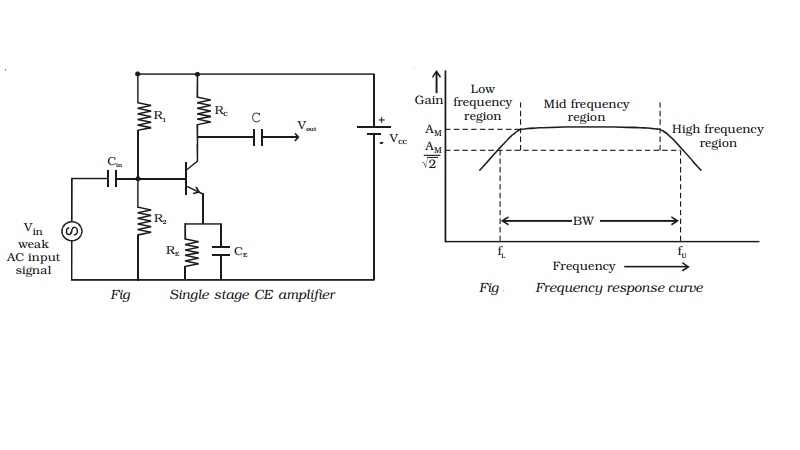Home | | Physics | | Physics | Single stage CE amplifier

# Single stage CE amplifierFig shows a single stage CE amplifier. The different circuit elements and their functions are described as follows. (i) Biasing circuit : The resistances R1, R2 and RE form the biasing and stabilization circuit.

Single stage CE amplifier

Fig  shows a single stage CE amplifier. The different circuit elements and their functions are described as follows.

(i) Biasing circuit : The resistances R1, R2 and RE form the biasing and stabilization circuit.

(ii)Input capacitance Cin : This is used to couple the signal to the base of the transistor. If this is not used, the signal source resistance will come across R2 and thus change the bias. The capacitor Cin allows only a.c. signal to flow.

(iii) Emitter bypass capacitor CE : This is connected in parallel with RE to provide a low reactance path to the amplified a.c. signal. If it is not used, then amplified a.c. signal flowing through RE will cause a voltage drop across it, thereby shifting the output voltage.

(iv)Coupling capacitor C : This is used to couple the amplified signal to the output device. This capacitor C allows only a.c. signal to flow.Working

When a weak input a.c. signal is applied to the base of the transistor, a small base current flows. Due to transistor action, a much larger a.c. current flows through collector load RC, a large voltage appears across RC and hence at the output. Therefore, a weak signal applied to the base appears in amplified form in the collector circuit. Voltage gain (Av) of the amplifier is the ratio of the amplified output voltage to the input voltage.

Frequency response and bandwidth

The voltage gain (Av) of the amplifier for different input frequencies can be determined. A graph can be drawn by taking frequency (f) along X-axis and voltage gain (Av) along Y-axis. The frequency response curve obtained will be of the form as shown in Fig. It can be seen that the gain decreases at very low and very high frequencies, but it remains constant over a wide range of mid-frequency region.

Lower cut off frequency (fL ) is defined as the frequency in the low 1frequency range at which the gain of the amplifier is 2 times the mid frequency gain (AM). Upper cut off frequency (fU) is defined as the frequency in the high frequency range at which the gain of the amplifier is 1/rt(2) times the mid frequency gain (AM).

Bandwidth is defined as the frequency interval between lower cut off and upper cut off frequencies.

BW = fU - fL

Study Material, Lecturing Notes, Assignment, Reference, Wiki description explanation, brief detail

Related Topics